# 二维变换

## 缩放$$x_1 = S_x \times x_0$$

$$y_1 = S_y \times y_0$$

$$\begin{bmatrix} x_1 \\ y_1 \end{bmatrix} = \begin{bmatrix} S_x & 0 \\ 0 & S_y \end{bmatrix} \begin{bmatrix} x_0 \\ y_0 \end{bmatrix}$$

## 旋转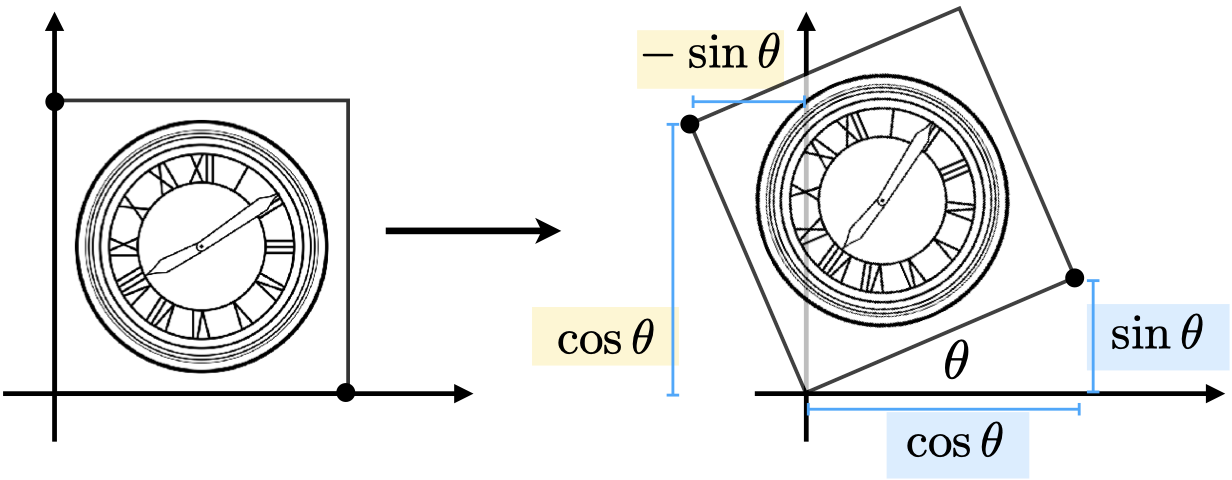$$\begin{bmatrix} x_1 \\ y_1 \end{bmatrix} = \begin{bmatrix} A & B \\ C & D \end{bmatrix} \begin{bmatrix} x_0 \\ y_0 \end{bmatrix}$$

$$\begin{bmatrix} cos \theta \\ sin \theta \end{bmatrix} = \begin{bmatrix} A & B \\ C & D \end{bmatrix} \begin{bmatrix} 1 \\ 0 \end{bmatrix}$$

$$cos \theta = A \times 1 + B \times 0 = A$$

$$sin \theta = C \times 1 + D \times 0 = C$$

$$\begin{bmatrix} -sin \theta \\ cos \theta \end{bmatrix} = \begin{bmatrix} A & B \\ C & D \end{bmatrix} \begin{bmatrix} 0 \\ 1 \end{bmatrix}$$

$$-sin \theta = A \times 0 + B \times 1 = B$$

$$cos \theta = C \times 0 + D \times 1 = D$$

$$R_\theta = \begin{bmatrix} cos \theta & -sin \theta \\ sin \theta & cos \theta \end{bmatrix}$$

## 线性变换

$$x_1 = ax_0 + by_0$$

$$y_1 = cx_0 + dy_0$$

$$\begin{bmatrix} x_1 \\ y_1 \end{bmatrix} = \begin{bmatrix} A & B \\ C & D \end{bmatrix} \begin{bmatrix} x_0 \\ y_0 \end{bmatrix}$$

## 平移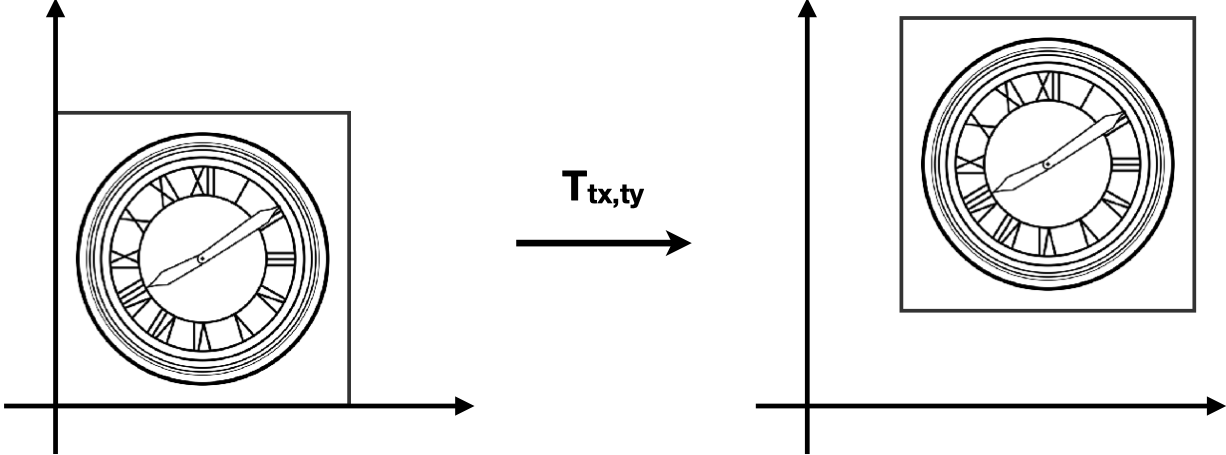$$x_1 = x_0 + T_x$$

$$y_1 = y_0 + T_y$$

## 齐次坐标

$$\begin{bmatrix} x_1 \\ y_1 \end{bmatrix} = \begin{bmatrix} A & B \\ C & D \end{bmatrix} \begin{bmatrix} x_0 \\ y_0 \end{bmatrix} + \begin{bmatrix} T_x \\ T_y \end{bmatrix}$$

• 2D 点： $(x, y, 1)$
• 2D 向量： $(x, y, 0)$

$$\begin{bmatrix} x_1 \\ y_1 \\ w_1 \end{bmatrix} = \begin{bmatrix} A & B & T_x \\ C & D & T_y \\ 0 & 0 & 1 \end{bmatrix} \begin{bmatrix} x_0 \\ y_0 \\ w_0 \end{bmatrix}$$

$$\begin{bmatrix} x_1 \\ y_1 \\ w_1 \end{bmatrix} = \begin{bmatrix} 1 & 0 & T_x \\ 0 & 1 & T_y \\ 0 & 0 & 1 \end{bmatrix} \begin{bmatrix} x_0 \\ y_0 \\ 0 \end{bmatrix} = \begin{bmatrix} x_0 + T_x \\ y_0 + T_y \\ 0 \end{bmatrix}$$

• 向量 + 向量 = 向量
• 因为 $w_1 + w_2 = 0$
• 点 - 点 = 向量
• 因为 $w_1 - w_2 = 0$
• 点 + 向量 = 点
• 因为 $w_1 + w_2 = 1$
• 点 + 点 = 这两点的中点
• 因为 $w_1 + w_2 = 2$，在齐次坐标下，点的 w 分量为 1，所以结果要除以 2，得到的结果正是两点的中点位置

## 逆变换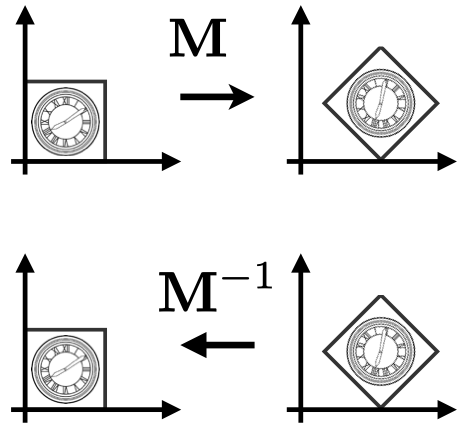## 仿射变换

$$仿射变换 = 线性变换 + 平移$$

$$\begin{bmatrix} x_1 \\ y_1 \end{bmatrix} = \begin{bmatrix} A & B \\ C & D \end{bmatrix} \begin{bmatrix} x_0 \\ y_0 \end{bmatrix} + \begin{bmatrix} T_x \\ T_y \end{bmatrix}$$

$$\begin{bmatrix} x_1 \\ y_1 \\ 1 \end{bmatrix} = \begin{bmatrix} A & B & T_x \\ C & D & T_y \\ 0 & 0 & 1 \end{bmatrix} \begin{bmatrix} x_0 \\ y_0 \\ 1 \end{bmatrix}$$

• 可以用一个矩阵来表示平移、旋转、缩放三种变换。
• 逆变换可以通过逆矩阵来表示。

$$T_{(x, y)} = \begin{bmatrix} 0 & 0 & T_x \\ 0 & 0 & T_y \\ 0 & 0 & 1 \end{bmatrix}$$

$$R_{(\theta)} = \begin{bmatrix} cos \theta & -sin \theta & 0 \\ sin \theta & cos \theta & 0 \\ 0 & 0 & 1 \end{bmatrix}$$

$$S_{(x, y)} = \begin{bmatrix} S_x & 0 & 0 \\ 0 & S_y & 0 \\ 0 & 0 & 1 \end{bmatrix}$$

## 组合变换

$$T \cdot R \cdot \begin{bmatrix} x \\ y \\ 1 \end{bmatrix}$$

$$A_n( \cdots A_2( A_1 \cdot \begin{bmatrix} x \\ y \\ 1 \end{bmatrix} ) = A_n \cdots A_2 \cdot A_1 \cdot \begin{bmatrix} x \\ y \\ 1 \end{bmatrix}$$

## 分解复杂变换

1. 把旋转中心平移到原点
2. 进行旋转
3. 把旋转中心平移回原来的位置

# 三维变换

• 3D 点表示为 $(x, y, z, 1)$
• 3D 向量表示为 $(x, y, z, 0)$

$$\begin{bmatrix} x_1 \\ y_1 \\ z_1 \\ 1 \end{bmatrix} = \begin{bmatrix} A & B & C & T_x \\ D & E & F & T_y \\ G & H & I & T_z \\ 0 & 0 & 0 & 1 \end{bmatrix} \begin{bmatrix} x_0 \\ y_0 \\ z_0 \\ 1 \end{bmatrix}$$

## 正交矩阵

$$R_\theta = \begin{bmatrix} cos \theta & -sin \theta \\ sin \theta & cos \theta \end{bmatrix}$$

$$R_{-\theta} = \begin{bmatrix} cos \theta & sin \theta \\ -sin \theta & cos \theta \end{bmatrix}$$

$${R_\theta}^T = \begin{bmatrix} cos \theta & sin \theta \\ -sin \theta & cos \theta \end{bmatrix}$$

$$R_{-\theta} = {R_\theta}^T$$

## 观测变换 (Viewing Transformation)

• 视图变换 (View Transformation)
• 投影变换 (Projection Transformation)
• 正交投影
• 透视投影

## 视图变换(世界空间)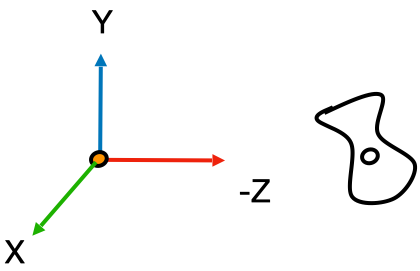(摄像机空间)

1. 摄像机的位置
2. 目标位置
3. 摄像机的上方向

1. 把摄像机移动到原点
2. 把摄像机的上方向调整成与 Y 一致，并让摄像机往 -Z 方向看

$$M_{view} = R_{view} \cdot T_{view}$$

$$T_{view} = \begin{bmatrix} 1 & 0 & 0 & -x \\\ 0 & 1 & 0 & -y \\\ 0 & 0 & 1 & -z \\\ 0 & 0 & 0 & 1 \end{bmatrix}$$

$$\hat e = \hat g \times \hat t$$

$${R_{view}}^{-1} \begin{bmatrix} x_{\hat g \times \hat t} & x_t & x_{-g} & 0 \\\ y_{\hat g \times \hat t} & y_t & y_{-g} & 0 \\\ z_{\hat g \times \hat t} & z_t & z_{-g} & 0 \\\ 0 & 0 & 0 & 1 \end{bmatrix}$$

$$R_{view} = {{R_{view}}^{-1}}^T = \begin{bmatrix} x_{\hat g \times \hat t} & y_{\hat g \times \hat t} & z_{\hat g \times \hat t} & 0 \\\ x_t & y_t & z_t & 0 \\\ x_{-g} & y_{-g} & z_{-g} & 0 \\\ 0 & 0 & 0 & 1 \end{bmatrix}$$

$$M_{view} = R_{view} \cdot T_{view} = \begin{bmatrix} x_{\hat g \times \hat t} & y_{\hat g \times \hat t} & z_{\hat g \times \hat t} & -x \\\ x_t & y_t & z_t & -y \\\ x_{-g} & y_{-g} & z_{-g} & -z \\\ 0 & 0 & 0 & 1 \end{bmatrix}$$

## 投影变换

• 正交投影（不会出现近大远小的现象）
• 透视投影（会出现近大远小的现象，透视投影下的平行线最终会汇聚在一点）

### 正交投影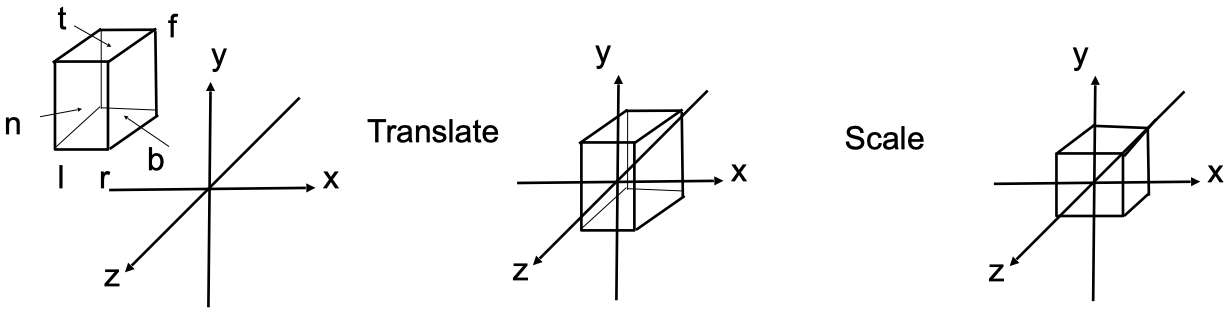• l = left
• r = right
• b = bottom
• t = top
• f = far
• n = near

1. 把空间平移到原点（空间的中心与原点重合）
2. 把空间压缩成 $[-1, 1]^3$

$$M_{ortho} = S_{ortho} \cdot T_{ortho}$$

$$T_{ortho} = \begin{bmatrix} 1 & 0 & 0 & -{\frac {l + r} 2} \\\ 0 & 1 & 0 & -{\frac {b + t} 2} \\\ 0 & 0 & 1 & -{\frac {f + n} 2} \\\ 0 & 0 & 0 & 1 \end{bmatrix}$$

$$(r - l) \times S_x = 2$$

$$S_x = \frac 2 {r - l}$$

$$S_{ortho} = \begin{bmatrix} \frac 2 {r - l} & 0 & 0 & 0 \\\ 0 & \frac 2 {t - b} & 0 & 0 \\\ 0 & 0 & \frac 2 {n - f} & 0 \\\ 0 & 0 & 0 & 1 \end{bmatrix}$$

$$M_{ortho} = S_{ortho} \cdot T_{ortho} = \begin{bmatrix} \frac 2 {r - l} & 0 & 0 & -{\frac {l + r} 2} \\\ 0 & \frac 2 {t - b} & 0 & -{\frac {b + t} 2} \\\ 0 & 0 & \frac 2 {n - f} & -{\frac {f + n} 2} \\\ 0 & 0 & 0 & 1 \end{bmatrix}$$

### 透视投影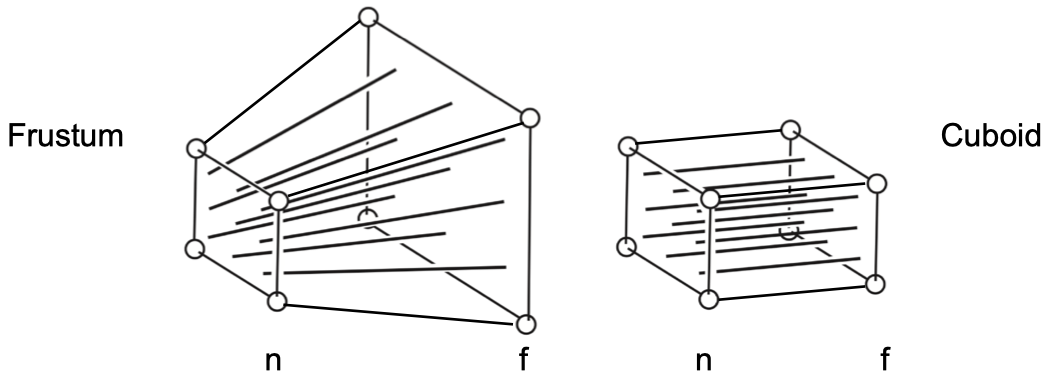1. 把空间平移到原点
2. 把空间压缩成长方体（近平面不变，压缩远平面）
3. 把空间压缩成 $[-1, 1]^3$ 的立方体（进行一次正交投影）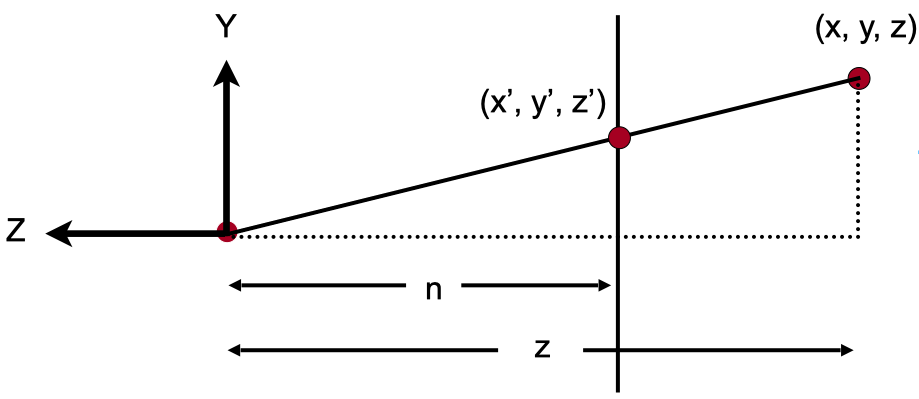$$\frac n z = \frac {y^{\prime}} y$$

$$y^{\prime} = \frac n z y$$

$$x^{\prime} = \frac n z x$$

$$M_{persp -> ortho} \begin{bmatrix} x \\\ y \\\ z \\\ 1 \end{bmatrix} = \begin{bmatrix} \frac {nx} z \\\ \frac {ny} z \\\ unknow \\\ 1 \end{bmatrix}$$

$$M_{persp -> ortho} \begin{bmatrix} x \\\ y \\\ z \\\ 1 \end{bmatrix} = \begin{bmatrix} nx \\\ ny \\\ unknow \\\ z \end{bmatrix}$$

$$ax + by + cz + d = nx$$

$$a = n$$

$$b = 0$$

$$c = 0$$

$$d = 0$$

$$M_{persp -> ortho} = \begin{bmatrix} n & 0 & 0 & 0 \\\ 0 & n & 0 & 0 \\\ ? & ? & ? & ? \\\ 0 & 0 & 1 & 0 \end{bmatrix}$$

• 任何在近平面的点，都不会发生变化，即：
$$\begin{bmatrix} ? & ? & ? & ? \end{bmatrix} \begin{bmatrix} x \\\ y \\\ n \\\ 1 \end{bmatrix} = \begin{bmatrix} x \\\ y \\\ n \\\ 1 \end{bmatrix} == \begin{bmatrix} nx \\\ ny \\\ n^2 \\\ n \end{bmatrix}$$

$$ax + by + cn + d = n^2$$

$$\begin{bmatrix} 0 & 0 & A & B \end{bmatrix}$$

• 任何在远平面上的店，z 都不会发生变化。我们取远平片中的一个中心点 $(0, 0, f, 1)$ ，它在空间压缩前后都不会发生变化，那么：
$$\begin{bmatrix} 0 & 0 & A & B \end{bmatrix} \begin{bmatrix} 0 \\\ 0 \\\ f \\\ 1 \end{bmatrix} = \begin{bmatrix} 0 \\\ 0 \\\ f \\\ 1 \end{bmatrix} == \begin{bmatrix} 0 \\\ 0 \\\ f^2 \\\ f \end{bmatrix}$$

$$An + B = n^2$$

$$Af + B = f^2$$

$$A = n + f$$

$$B = -nf$$

$$M_{persp -> ortho} = \begin{bmatrix} n & 0 & 0 & 0 \\\ 0 & n & 0 & 0 \\\ 0 & 0 & n + f & -nf \\\ 0 & 0 & 1 & 0 \end{bmatrix}$$

$$M_{persp} = M_{ortho} M_{persp -> ortho}$$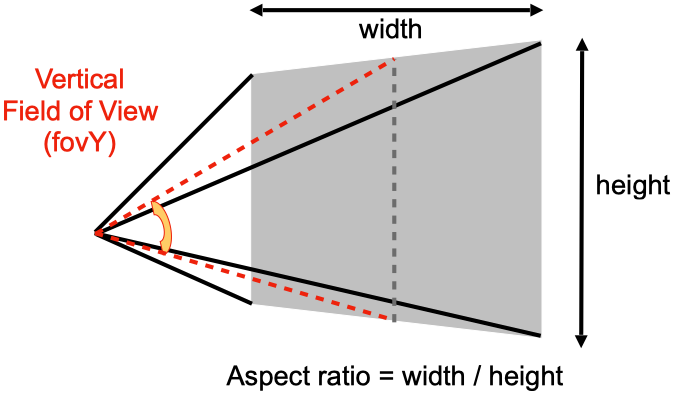fov 是指视野范围，分为 fovYfovX，两者可以相互推导。

aspect ratio 是指近平面的宽高比。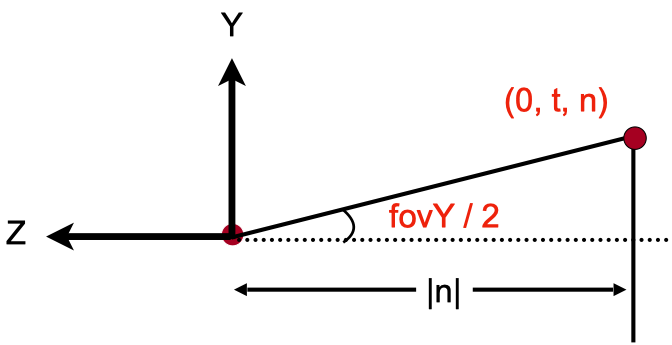$$tan {\frac {fovY} 2} = \frac t {|n|}$$

$$aspect = \frac {width} {height} = \frac {2r} {2t} = \frac r t$$

$$t = |n| \cdot tan {\frac {fovY} 2}$$

$$r = aspect \cdot t$$

$$b = -t$$

$$l = -r$$

# 作业

## 基础

  1 2 3 4 5 6 7 8 9 10 11 12 13 14 15 16 17 18 19 20 21 22 23 24 25 26 27 28 29 30 31 32 33 34 35 36 37 38 39 40 41 42 43 44 45 46 47 48 49 50 51 52 53 54 55 56  // main.cpp #include constexpr double MY_PI = 3.1415926; constexpr double DEG_TO_RAD = MY_PI / 180.0; Eigen::Matrix4f get_model_matrix(float rotation_angle) { Eigen::Matrix4f model = Eigen::Matrix4f::Identity(); float rad = rotation_angle * DEG_TO_RAD; float sin_theta = sin(rad); float cos_theta = cos(rad); model(0, 0) = cos_theta; model(0, 2) = -sin_theta; model(2, 0) = sin_theta; model(2, 2) = cos_theta; return model; } Eigen::Matrix4f get_projection_matrix(float eye_fov, float aspect_ratio, float zNear, float zFar) { Eigen::Matrix4f projection = Eigen::Matrix4f::Identity(); Eigen::Matrix4f ortho = Eigen::Matrix4f::Identity(); Eigen::Matrix4f persp_to_ortho = Eigen::Matrix4f::Identity(); float n = zNear; float f = zFar; float t = n * tan((eye_fov / 2.0) * DEG_TO_RAD); float r = aspect_ratio * t; float b = -t; float l = -r; ortho(0, 0) = 2 / (r - l); ortho(1, 1) = 2 / (t - b); ortho(2, 2) = 2 / (n - f); ortho(0, 3) = -(l + r) / 2; ortho(1, 3) = -(b + t) / 2; ortho(2, 3) = -(f + n) / 2; persp_to_ortho(0, 0) = n; persp_to_ortho(1, 1) = n; persp_to_ortho(2, 2) = n + f; persp_to_ortho(2, 3) = -(n * f); persp_to_ortho(3, 2) = 1; persp_to_ortho(3, 3) = 0; projection = ortho * persp_to_ortho; return projection; } 

# 参考资料

Lecture 03 Transformation

Lecture 04 Transformation Cont.

Lecture 05 Rasterization 1 (Triangles)

Rodrigues 旋轉公式